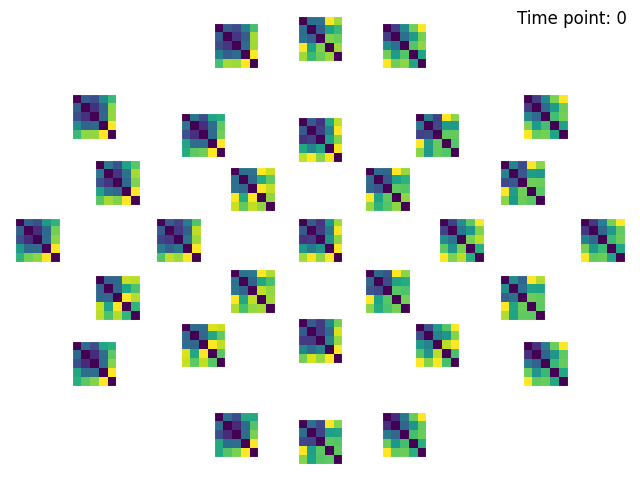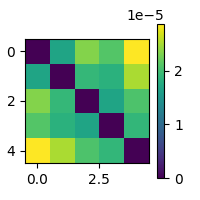# Using cross-validation when computing DSMs¶

This example demonstrates how to perform cross-validation when computing dissimilarity matrices (DSMs). When the data has repeated measurements of the same stimulus type, cross-validation can be used to provide much more robust distance estimates between stimulus types. Repeated measurements can for example be actual repetitions of the same stimulus within the same recording, or recordings on multiple volunteers with the same stimuli.

The dataset will be the kiloword dataset : approximately 1,000 words were presented to 75 participants in a go/no-go lexical decision task while event-related potentials (ERPs) were recorded.

This dataset as provided does not have repeated measurements of the same stimuli. To illustrate cross-validation, we will treat words with the same number of letters as being repeated measurements of the same stimulus type.

```# Import required packages
import mne
import mne_rsa
```

MNE-Python contains a build-in data loader for the kiloword dataset. We use it here to read it as 960 epochs. Each epoch represents the brain response to a single word, averaged across all the participants. For this example, we speed up the computation, at a cost of temporal precision, by downsampling the data from the original 250 Hz. to 100 Hz.

```data_path = mne.datasets.kiloword.data_path(verbose=True)
epochs = epochs.resample(100)
```
```Reading C:\Users\wmvan\mne_data\MNE-kiloword-data\kword_metadata-epo.fif ...
Found the data of interest:
t =    -100.00 ...     920.00 ms
0 CTF compensation matrices available
960 matching events found
No baseline correction applied
0 projection items activated
```

The `epochs` object contains a `.metadata` field that contains information about the 960 words that were used in the experiment. Let’s have a look at the metadata for the 10 random words:

```epochs.metadata.sample(10)
```
WORD Concreteness WordFrequency OrthographicDistance NumberOfLetters BigramFrequency ConsonantVowelProportion VisualComplexity
552 stern 3.650000 2.190332 1.90 5.0 549.800000 0.800000 60.215695
19 pale 4.450000 3.004751 1.00 4.0 732.500000 0.500000 69.970377
130 sleeve 5.850000 2.230449 2.00 6.0 305.333333 0.500000 67.415729
883 promise 2.750000 2.704151 2.10 7.0 702.857143 0.571429 68.739179
571 quirk 2.947368 1.255273 1.85 5.0 206.600000 0.600000 59.921672
678 interest 2.550000 3.582745 2.40 8.0 1242.625000 0.625000 58.784077
887 victory 2.950000 2.881955 2.55 7.0 360.000000 0.571429 55.360843
649 triumph 3.100000 2.670246 2.75 7.0 361.428571 0.714286 63.660586
521 lava 6.050000 1.806180 1.85 4.0 275.750000 0.500000 62.325214
267 dash 4.100000 2.012837 1.45 4.0 398.250000 0.750000 75.452526

The kiloword dataset as provided does not have repeated measurements of the same stimuli. To illustrate cross-validation, we will treat words with the same number of letters as being repeated measurements of the same stimulus type.

To denote which epochs are repetitions of the same stimulus, we create a list `y` that contains integer labels for each epoch. Repetitions of the same stimulus have the same label in the `y` list. This scheme is lifted from the machine learning literature (and the Scikit-Learn API). In this example, we use the number of letters in the stimulus words as the labels for the epochs.

```y = epochs.metadata.NumberOfLetters.astype(int)
```

Many high-level functions in the MNE-RSA module can take the `y` list as a parameter to enable cross-validation. Notably the functions for performing RSA and computing DSMs. In this example, we will restrict the analysis to computing DSMs using a spatio-temporal searchlight on the sensor-level data.

```dsms = mne_rsa.dsm_epochs(
epochs,                      # The EEG data
y=y,                         # Set labels to enable cross validation
n_folds=5,                   # Number of folds to use during cross validation
dist_metric='sqeuclidean',   # Distance metric to compute the DSMs
tmin=0.15, tmax=0.25)        # To save time, only analyze this time interval
```

Plotting the cross-validated DSMs

```mne_rsa.plot_dsms_topo(dsms, epochs.info)
``````Creating spatio-temporal searchlight patches

<Figure size 640x480 with 29 Axes>
```

For performance reasons, the low-level functions of MNE-RSA do not take a `y` list for cross-validation. Instead, they require the data to be already split into folds. The `create_folds()` function can create these folds.

```X = epochs.get_data()```<Figure size 200x200 with 2 Axes>